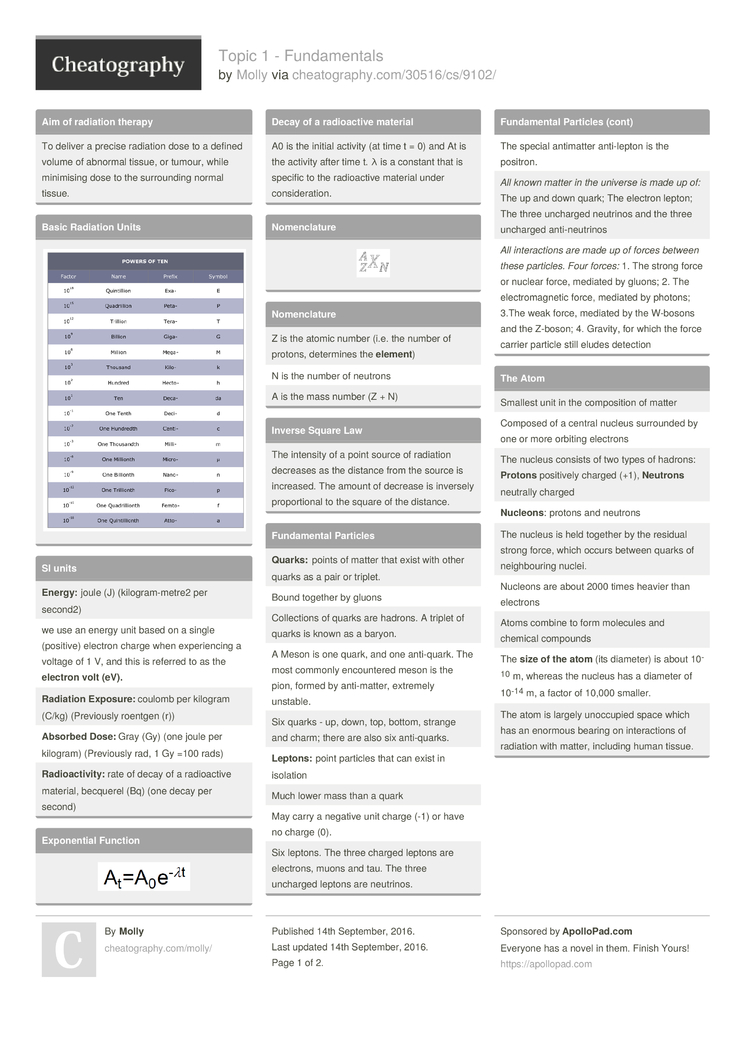# Topic 1 - Fundamentals by Molly

### Basic Radiation Units### SI units

 Ener­gy: joule (J) (kilog­ram­-metre2 per second2) we use an energy unit based on a single (positive) electron charge when experi­encing a voltage of 1 V, and this is referred to as the electron volt (eV). Radi­ation Exposu­re: coulomb per kilogram (C/kg) (Previ­ously roentgen (r)) Absorbed Dose: Gray (Gy) (one joule per kilogram) (Previ­ously rad, 1 Gy =100 rads) Radi­oac­tiv­ity: rate of decay of a radioa­ctive material, becquerel (Bq) (one decay per second)

### Expone­ntial Function### Decay of a radioa­ctive material

 A0 is the initial activity (at time t = 0) and At is the activity after time t. λ is a constant that is specific to the radioa­ctive material under consid­era­tion.

### Nomenc­lature### Nomenc­lature

 Z is the atomic number (i.e. the number of protons, determines the elem­ent) N is the number of neutrons A is the mass number (Z + N)

### Inverse Square Law

 The intensity of a point source of radiation decreases as the distance from the source is increased. The amount of decrease is inversely propor­tional to the square of the distance.

### Fundam­ental Particles

 Quar­ks: points of matter that exist with other quarks as a pair or triplet. Bound together by gluons Collec­tions of quarks are hadrons. A triplet of quarks is known as a baryon. A Meson is one quark, and one anti-q­uark. The most commonly encoun­tered meson is the pion, formed by anti-m­atter, extremely unstable. Six quarks - up, down, top, bottom, strange and charm; there are also six anti-q­uarks. Lept­ons: point particles that can exist in isolation Much lower mass than a quark May carry a negative unit charge (-1) or have no charge (0). Six leptons. The three charged leptons are electrons, muons and tau. The three uncharged leptons are neutrinos. The special antimatter anti-l­epton is the positron. All known matter in the universe is made up of: The up and down quark; The electron lepton; The three uncharged neutrinos and the three uncharged anti-n­eut­rinos All intera­ctions are made up of forces between these particles. Four forces: 1. The strong force or nuclear force, mediated by gluons; 2. The electr­oma­gnetic force, mediated by photons; 3.The weak force, mediated by the W-bosons and the Z-boson; 4. Gravity, for which the force carrier particle still eludes detection

### The Atom

 Smallest unit in the compos­ition of matter Composed of a central nucleus surrounded by one or more orbiting electrons The nucleus consists of two types of hadrons: Prot­ons positively charged (+1), Neut­rons neutrally charged Nucl­eons: protons and neutrons The nucleus is held together by the residual strong force, which occurs between quarks of neighb­ouring nuclei. Nucleons are about 2000 times heavier than electrons Atoms combine to form molecules and chemical compounds The size of the atom (its diameter) is about 10-10 m, whereas the nucleus has a diameter of 10-14 m, a factor of 10,000 smaller. The atom is largely unoccupied space which has an enormous bearing on intera­ctions of radiation with matter, including human tissue.

### The Electron Position

 Heis­enb­erg's Uncert­ainty Princi­ple: the exact momentum (energy) and the exact position can't be known simult­ane­ously Observing something alters it

### Wave-P­article Duality

 We can consider the atomic entity as either a: 1. part­icle (the localised ‘billiard ball’ approach) with particle diameter (d) and mass (m) 2. wave (an extended and vibrating phenom­enon) with energy (E), wavelength (λ), and frequency (f). E = mc2 Energy here is in J and must be converted into MeV.

### Atomic Mass Unit (u)

 One atomic mass unit is 1/12 of the mass of the carbon-12 atom.

### Isotopes

 Elements exist with different numbers of neutrons than the neutral atom 'Isotope’ does not necess­arily imply a radioa­ctive material.

### Electronic Structure of the Atom

 Bohr model: electrons rotate around the nucleus in discrete energy shells that are stationary and arranged in increasing order of energy. A maximum number of electrons allowable in each shell K shell can hold 2 electrons, the L shell 8 electrons, the M shell 18 electrons, etc. Orbital electrons don't actually exist in precise circular orbits, but rather in imprec­isely defined regions of space around the nucleus The electron’s position is defined by probab­ility, with decreasing probab­ility for locations outside of the ‘most likely’ regions

### Electron Binding Energy

 Electrons have different binding energies, depending on the electron shell In the most stable config­ura­tions, electrons occupy the innermost shells where they are most tightly bound to the nucleus. Exci­tat­ion: an electron is raised from a lower energy shell to an upper energy shell (releasing energy) Ioni­sat­ion: an electron is removed completely from an atom Binding energy of an electron is the energy required to remove it completely from a shell Binding energy is higher for orbitals nearer the nucleus (KB>LB­>MB). Binding energy increases with the charge (equal to the atomic number Z) of the nucleus Removing an electr­on/­going from an inner to an outer shell, requires energy input An electron moving from an outer to an inner shell results in energy emission

## Download the Topic 1 - Fundamentals2 Pages
//media.cheatography.com/storage/thumb/molly_topic-1-fundamentals.750.jpg

PDF (recommended)